Updating search results...

# 38 Results

View
Selected filters:
• CCSS.Math.Content.6.EE.A.2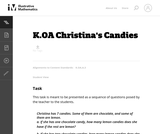Unrestricted Use
CC BY
Rating
0.0 stars

The purpose of this task is for students to find different pairs of numbers that sum to 7.

Subject:
Mathematics
Measurement and Data
Material Type:
Activity/Lab
Provider:
Illustrative Mathematics
Provider Set:
Illustrative Mathematics
Author:
Illustrative Mathematics
05/01/2012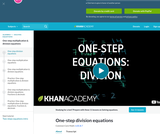Rating
0.0 stars

Khan Academy Video Tutorial. Introduction to basic algebraic equations of the form Ax=B

Subject:
Education
Mathematics
Algebra
Material Type:
Teaching/Learning Strategy
Provider:
Florida Center for Research in Science, Technology, Engineering, and Mathematics
Provider Set:
iCPALMS: A Standards-based K-12 Resources and Tools Pathway
Author:
Salman Khan
04/07/2011Conditional Remix & Share Permitted
CC BY-NC
Rating
5.0 stars

This unit is an EQuIP Exemplar for adult education (http://achieve.org/equip). Students will connect their prior, real-world knowledge to the concept of order in mathematics. They will go through a discovery process with content that will build a deep, conceptual understanding of the properties of operations to explain why we perform operations in a certain order when we see just the naked numbers.

Material Type:
Lesson Plan
Unit of Study
Provider:
EQuIP Exemplars
Author:
Connie Rivera
11/26/2014Only Sharing Permitted
CC BY-NC-ND
Rating
4.0 stars

This lesson unit is intended to help you assess how well students are able to: Perform arithmetic operations, including those involving whole-number exponents, recognizing and applying the conventional order of operations; Write and evaluate numerical expressions from diagrammatic representations and be able to identify equivalent expressions; apply the distributive and commutative properties appropriately; and use the method for finding areas of compound rectangles.

Subject:
Geometry
Material Type:
Assessment
Lesson Plan
Provider:
Shell Center for Mathematical Education
Provider Set:
Mathematics Assessment Project (MAP)
04/26/2013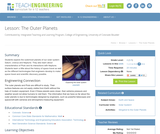Educational Use
Rating
0.0 stars

Students explore the outermost planets of our solar system: Saturn, Uranus and Neptune. They also learn about characteristics of Pluto and its interactions with Neptune. Students learn a little about the history of space travel as well as the different technologies that engineers develop to make space travel and scientific discovery possible.

Subject:
Engineering
Astronomy
Material Type:
Activity/Lab
Lesson Plan
Provider:
TeachEngineering
Provider Set:
TeachEngineering
Author:
Denise W. Carlson
Jessica Butterfield
Jessica Todd
Malinda Schaefer Zarske
Sam Semakula
09/18/2014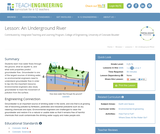Educational Use
Rating
0.0 stars

Groundwater is one of the largest sources of drinking water, so environmental engineers need to understand groundwater flow in order to tap into this important resource. Environmental engineers also study groundwater to predict where pollution from the surface may end up. In this lesson, students will learn how water flows through the ground, what an aquifer is and what soil properties are used to predict groundwater flow.

Subject:
Engineering
Hydrology
Material Type:
Activity/Lab
Lesson Plan
Provider:
TeachEngineering
Provider Set:
TeachEngineering
Author:
Janet Yowell
Malinda Schaefer Zarske
Melissa Straten
09/18/2014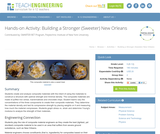Educational Use
Rating
0.0 stars

Students create and analyze composite materials with the intent of using the materials to construct a structure with optimal strength and minimal density. The composite materials are made of puffed rice cereal, marshmallows and chocolate chips. Student teams vary the concentrations of the three components to create their composite materials. They determine the material density and test its compressive strength by placing weights on it and measuring how much the material compresses. Students graph stress vs. strain and determine Young's modulus to analyze the strength of their materials.

Subject:
Architecture and Design
Engineering
Material Type:
Activity/Lab
Provider:
TeachEngineering
Provider Set:
TeachEngineering
Author:
Charisse Nelson
Sarah Wigodsky
10/14/2015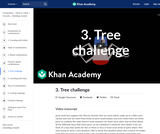Conditional Remix & Share Permitted
CC BY-NC-SA
Rating
0.0 stars

How can trees be combined to count robots with many parts?

Subject:
Applied Science
Computer Science
Graphic Arts
Material Type:
Lesson
Provider:
Provider Set:
Pixar
Author:
Disney Pixar
07/14/2021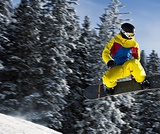Conditional Remix & Share Permitted
CC BY-NC
Rating
0.0 stars

Students do a card sort in which they match expressions in words with their equivalent algebraic expressions.Key ConceptsA mathematical expression that uses letters to represent numbers is an algebraic expression.A letter used in place of a number in an expression is called a variable.An algebraic expression combines both numbers and letters using the arithmetic operations of addition (+), subtraction (–), multiplication (·), and division (÷) to express a quantity.Words can be used to describe algebraic expressions.There are conventions for writing algebraic expressions:The product of a number and a variable lists the number first with no multiplication sign. For example, the product of 5 and n is written as 5n, not n5.The product of a number and a factor in parentheses lists the number first with no multiplication sign. For example, write 5(x + 3), not (x + 3)5.For the product of 1 and a variable, either write the multiplication sign or do not write the "1." For example, the product of 1 and z is written either 1 ⋅ z or z, not 1z.Goals and Learning ObjectivesTranslate between expressions in words and expressions in symbols.

Subject:
Algebra
Material Type:
Lesson Plan
Provider:
Pearson
09/21/2015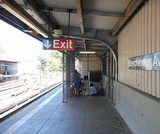Conditional Remix & Share Permitted
CC BY-NC
Rating
0.0 stars

Students critique the work of other students and revise their own work based on feedback from the teacher and peers.Key ConceptsConcepts from previous lessons are integrated into this unit task: rewriting expressions, using parentheses, and using the distributive property. Students apply their knowledge, review their work, and make revisions based on feedback from you and their peers. This process creates a deeper understanding of the concepts.Goals and Learning ObjectivesApply knowledge of expressions to correct the work of other students.Track and review the choice of strategy when problem solving.

Subject:
Mathematics
Material Type:
Lesson Plan
Provider:
Pearson
09/21/2015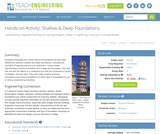Educational Use
Rating
0.0 stars

Students investigate the critical nature of foundations as they learn differences between shallow and deep foundations, including the concepts of bearing pressure and settlement. Using models representing a shallow foundation and a deep pile foundation, they test, see and feel the effects in a cardboard box test bed composed of layers of pebbles, soil and sand. They also make bearing pressure calculations and recommendations for which type of foundations to use in various engineering scenarios.

Subject:
Education
Material Type:
Activity/Lab
Provider:
TeachEngineering
Provider Set:
TeachEngineering
Author:
Denali Lander
Denise W. Carlson
Joe Friedrichsen
Jonathan S. Goode
Malinda Schaefer Zarske
Natalie Mach
10/14/2015Conditional Remix & Share Permitted
CC BY-NC
Rating
0.0 stars

Subject:
Mathematics
Material Type:
Full Course
Provider:
Pearson
10/06/2016Rating
0.0 stars

Getting Started

Type of Unit: Introduction

Prior Knowledge

Students should be able to:

Solve and write numerical equations for whole number addition, subtraction, multiplication, and division problems.
Use parentheses to evaluate numerical expressions.
Identify and use the properties of operations.

Lesson Flow

In this unit, students are introduced to the rituals and routines that build a successful classroom math community and they are introduced to the basic features of the digital course that they will use throughout the year.

An introductory card sort activity matches students with their partner for the week. Then over the course of the week, students learn about the lesson routines: Opening, Work Time, Ways of Thinking, Apply the Learning, Summary of the Math, and Reflection. Students learn how to present their work to the class, the importance of taking responsibility for their own learning, and how to effectively participate in the classroom math community.

Students then work on Gallery problems to further explore the program’s technology resources and tools and learn how to organize their work.

The mathematical work of the unit focuses on numerical expressions, including card sort activities in which students identify equivalent expressions and match an expression card to a word card that describes its meaning. Students use the properties of operations to identify equivalent expressions and to find unknown values in equations.

Subject:
Mathematics
Material Type:
Unit of Study
Provider:
PearsonRating
0.0 stars

Expressions

Type of Unit: Concept

Prior Knowledge

Students should be able to:

Write and evaluate simple expressions that record calculations with numbers.
Use parentheses, brackets, or braces in numerical expressions and evaluate expressions with these symbols.
Interpret numerical expressions without evaluating them.

Lesson Flow

Students learn to write and evaluate numerical expressions involving the four basic arithmetic operations and whole-number exponents. In specific contexts, they create and interpret numerical expressions and evaluate them. Then students move on to algebraic expressions, in which letters stand for numbers. In specific contexts, students simplify algebraic expressions and evaluate them for given values of the variables. Students learn about and use the vocabulary of algebraic expressions. Then they identify equivalent expressions and apply properties of operations, such as the distributive property, to generate equivalent expressions. Finally, students use geometric models to explore greatest common factors and least common multiples.

Subject:
Mathematics
Algebra
Material Type:
Unit of Study
Provider:
Pearson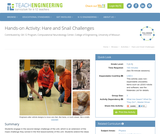Educational Use
Rating
0.0 stars

Students engage in the second design challenge of the unit, which is an extension of the maze challenge they solved in the first lesson/activity of this unit. Students extend the ideas learned in the maze challenge with a focus more on the robot design. Gears are a very important part of any machine, particularly when it has a power source such as engine or motor. Specifically, students learn how to design the gear train from the LEGO MINDSTORMS(TM) NXT servomotor to the wheel to make the LEGO taskbot go faster or slower. A PowerPoint® presentation, pre/post quizzes and a worksheet are provided.

Subject:
Engineering
Electronic Technology
Material Type:
Activity/Lab
Provider:
TeachEngineering
Provider Set:
TeachEngineering
Author:
Pranit Samarth
Sachin Nair
Satish S. Nair
09/18/2014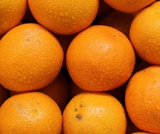Conditional Remix & Share Permitted
CC BY-NC
Rating
0.0 stars

Students are introduced to classroom routines and expectations, and complete a full mathematics lesson. The class discusses how to clearly present work to classmates. Partner work is modeled, and partners then work to match numerical expressions to corresponding word descriptions. Students read and discuss a summary of the math in the lesson, and then write a reflection about their thoughts.Key ConceptsStudents match a numerical expression to its corresponding description in words. Students interpret parentheses and brackets in numerical expressions and they construct viable arguments and critique the reasoning of others. Students learn to use the exponent 2 to represent squaring.Goals and Learning ObjectivesDescribe the classroom routines and expectations.Consider how to present work clearly to classmates.Collaborate with a partner.Critique a partner’s reasoning.Connect a numerical expression to its corresponding word description.Learn to use an exponent of 2 to represent squaring.

Subject:
Algebra
Material Type:
Lesson Plan
Provider:
Pearson
09/21/2015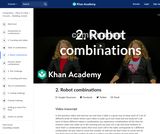Conditional Remix & Share Permitted
CC BY-NC-SA
Rating
0.0 stars

Let's review the multiplication principle which allows us to quickly count the number of possible robots.

Subject:
Applied Science
Computer Science
Graphic Arts
Material Type:
Lesson
Provider:
Provider Set:
Pixar
Author:
Disney Pixar
07/14/2021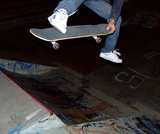Conditional Remix & Share Permitted
CC BY-NC
Rating
0.0 stars

Students play an Expressions Game in which they describe expressions to their partners using the vocabulary of expressions: term, coefficient, exponent, constant, and variable. Their partners try to write the correct expressions based on the descriptions.Key ConceptsMathematical expressions have parts, and these parts have names. These names allow us to communicate with others in a precise way.A variable is a symbol (usually a letter) in an expression that can be replaced by a number.A term is a number, a variable, or a product of numbers and variables. Terms are separated by the operator symbols + (plus) and – (minus).A coefficient is a symbol (usually a number) that multiplies the variable in an algebraic expression.An exponent tells how many copies of a number or variable are multiplied together.A constant is a number. In an expression, it can be a constant term or a constant coefficient. In the expression 2x + 3, 2 is a constant coefficient and 3 is a constant term.Goals and Learning ObjectivesIdentify parts of an expression using appropriate mathematical vocabulary.Write expressions that fit specific descriptions (for example, the expression is the sum of two terms each with a different variable).

Subject:
Algebra
Material Type:
Lesson Plan
Provider:
Pearson
09/21/2015Conditional Remix & Share Permitted
CC BY-NC-SA
Rating
0.0 stars

Let's get to know Fran Kalal.

Subject:
Applied Science
Computer Science
Graphic Arts
Material Type:
Lesson
Provider:
Provider Set:
Pixar
Author:
Disney Pixar
07/14/2021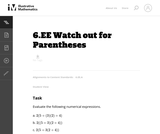Unrestricted Use
CC BY
Rating
0.0 stars

This problem asks the student to evaluate three numerical expressions that contain the same integers yet have differing results due to placement of parentheses.

Subject:
Mathematics
Material Type:
Activity/Lab
Provider:
Illustrative Mathematics
Provider Set:
Illustrative Mathematics
Author:
Illustrative Mathematics